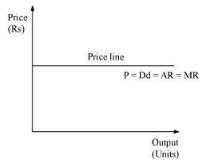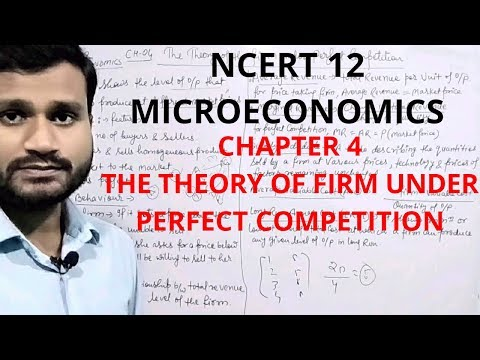Courses

# Test: Theory Of The Firm Under Perfect Competition - 2

## 10 Questions MCQ Test Economics Class 12 | Test: Theory Of The Firm Under Perfect Competition - 2

Description
This mock test of Test: Theory Of The Firm Under Perfect Competition - 2 for UPSC helps you for every UPSC entrance exam. This contains 10 Multiple Choice Questions for UPSC Test: Theory Of The Firm Under Perfect Competition - 2 (mcq) to study with solutions a complete question bank. The solved questions answers in this Test: Theory Of The Firm Under Perfect Competition - 2 quiz give you a good mix of easy questions and tough questions. UPSC students definitely take this Test: Theory Of The Firm Under Perfect Competition - 2 exercise for a better result in the exam. You can find other Test: Theory Of The Firm Under Perfect Competition - 2 extra questions, long questions & short questions for UPSC on EduRev as well by searching above.
QUESTION: 1

### This a MCQ (Multiple Choice Question) based practice test of Chapter 4 - Theory of Firm Under Perfect Competition of Economics of Class XII (12) for the quick revision/preparation of School Board examinations Q  Condition for producer equilibrium is:

Solution:

Producer's Equilibrium: Equilibrium refers to a state of rest when no change is required. A firm (producer) is said to be in equilibrium when it has no inclination to expand or to contract its output. This state either reflects maximum profits or minimum losses. Producer’s equilibrium is often explained in terms of marginal revenue (MR) and marginal cost (MC) of production. Profit is maximized (or a producer strikes his equilibrium) when two conditions are satisfied – (i) MR = MC, and (ii) MC is rising (or MC is greater than MR beyond the point of equilibrium output)

QUESTION: 2

### For maximum profit, the condition is:

Solution:

This strategy is based on the fact that the total profit reaches its maximum point where marginal revenue equals marginal profit. This is the case because the firm will continue to produce until marginal profit is equal to zero, and marginal profit equals the marginal revenue (MR) minus the marginal cost (MC).

QUESTION: 3

### ____________ is an ideal market?

Solution:

Pure or perfect competition is a theoretical market structure in which the following criteria are met: All firms sell an identical product (the product is a "commodity" or "homogeneous"). All firms are price takers (they cannot influence the market price of their product). Market share has no influence on prices.

QUESTION: 4

Under which market situation demand curve is linear and parallel to X-axis?

Solution:
QUESTION: 5

For a monopolist, the necessary condition for equilibrium is?

Solution:

In a monopolistic market, there is only one firm that produces a product. There is absolute product differentiation because there is no substitute. The marginal cost of production is the change in the total cost that arises when there is a change in the quantity produced. The marginal revenue is the change in the total revenue that arises when there is a change in the quantity produced. a firm maximizes its total profit by equating marginal cost to marginal revenue and solving for the price of one product and the quantity it must produce.

QUESTION: 6

Supernormal profit occur, when?

Solution:

Supernormal profit is defined as extra profit above that level of normal profit. Supernormal profit is also known as abnormal profit. Abnormal profit means there is an incentive for other firms to enter the industry.

QUESTION: 7

MR curve=AR=Demand curve is a feature of which kind of market?

Solution:
QUESTION: 8

If under perfect competition, the price lies below the average cost curve, the firm would?

Solution:
QUESTION: 9

What are the conditions for the long run equilibrium of the competitive firm?

Solution:

For a firm to achieve long run equilibrium, the marginal cost must be equal to the price and the long run average cost. That is, LMC = LAC = P. The firm adjusts the size of its plant to produce a level of output at which the LAC is minimum.

QUESTION: 10

Under which market condition firms make only Normal Profit in the long run?

Solution:

Monopolistic competition is a market structure which combines elements of monopoly and competitive markets. Essentially a monopolistic competitive market is one with freedom of entry and exit, but firms can differentiate their products. Because there is freedom of entry, supernormal profits will encourage more firms to enter the market leading to normal profits in the long term.

Track your progress, build streaks, highlight & save important lessons and more!

### Similar Content### Related tests